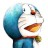# 杰拉斯的博客

## [ACM_ZOJ_1733]Longest Common Subsequence杰拉斯 | 时间：2012-04-04, Wed | 40,094 views

### Common Subsequence （最长公共子序列）

Time Limit: 2 Seconds Memory Limit: 65536 KB

#### Description

A subsequence of a given sequence is the given sequence with some elements (possible none) left out. Given a sequence X = another sequence Z = is a subsequence of X if there exists a strictly increasing sequence of indices of X such that for all j = 1,2,...,k, xij = zj. For example, Z = is a subsequence of X = with index sequence . Given two sequences X and Y the problem is to find the length of the maximum-length common subsequence of X and Y.

The program input is from a text file. Each data set in the file contains two strings representing the given sequences. The sequences are separated by any number of white spaces. The input data are correct. For each set of data the program prints on the standard output the length of the maximum-length common subsequence from the beginning of a separate line.

(阅读全文…)

## [ACM_POJ_2533]Longest Ordered Subsequence杰拉斯 | 时间：2012-04-03, Tue | 19,852 views

### Longest Ordered Subsequence （最长有序子序列）

Time Limit: 2000MS Memory Limit: 65536K
Total Submissions: 21942 Accepted: 9441

#### Description

A numeric sequence of ai is ordered if a1 < a2 < ... < aN. Let the subsequence of the given numeric sequence (a1, a2, ..., aN) be any sequence (ai1, ai2, ..., aiK), where 1

Your program, when given the numeric sequence, must find the length of its longest ordered subsequence.

#### Input

The first line of input file contains the length of sequence N. The second line contains the elements of sequence - N integers in the range from 0 to 10000 each, separated by spaces. 1 <= N <= 1000

#### Output

Output file must contain a single integer - the length of the longest ordered subsequence of the given sequence.

(阅读全文…)

## [ACM_ZJUT_1029]斐波那契数列杰拉斯 | 时间：2012-04-03, Tue | 17,159 views

### Fibonacci数

Time Limit:1000MS Memory Limit:32768K

```5
6
7
8
9
40
```

```5
8
13
21
34
102334155
```

(阅读全文…)

## [ACM_POJ_2081]动态规划入门练习（三）Recaman's Sequence杰拉斯 | 时间：2012-04-03, Tue | 5,411 views

### Recaman's Sequence

Time Limit: 3000MS Memory Limit: 60000K
Total Submissions: 17939 Accepted: 7450

#### Description

The Recaman's sequence is defined by a0 = 0 ; for m > 0, am = am−1 − m if the rsulting am is positive and not already in the sequence, otherwise am = am−1 + m.
The first few numbers in the Recaman's Sequence is 0, 1, 3, 6, 2, 7, 13, 20, 12, 21, 11, 22, 10, 23, 9 ...

#### Input

The input consists of several test cases. Each line of the input contains an integer k where 0 <= k <= 500000.
The last line contains an integer −1, which should not be processed.

#### Output

For each k given in the input, print one line containing ak to the output.

(阅读全文…)

## [ACM_POJ_1579]动态规划入门练习（二）Function Run Fun杰拉斯 | 时间：2012-04-03, Tue | 21,571 views

### Function Run Fun

Time Limit: 1000MS Memory Limit: 10000K
Total Submissions: 12362 Accepted: 6466

#### Description

We all love recursion! Don't we?

Consider a three-parameter recursive function w(a, b, c):

if a <= 0 or b <= 0 or c <= 0, then w(a, b, c) returns: 1 if a > 20 or b > 20 or c > 20, then w(a, b, c) returns:
w(20, 20, 20)

if a < b and b < c, then w(a, b, c) returns:
w(a, b, c-1) + w(a, b-1, c-1) - w(a, b-1, c)

otherwise it returns:
w(a-1, b, c) + w(a-1, b-1, c) + w(a-1, b, c-1) - w(a-1, b-1, c-1)

This is an easy function to implement. The problem is, if implemented directly, for moderate values of a, b and c (for example, a = 15, b = 15, c = 15), the program takes hours to run because of the massive recursion.

(阅读全文…)